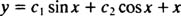## Second-Order Homogeneous Equations

There are two definitions of the term “homogeneous differential equation.” One definition calls a first‐order equation of the form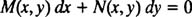homogeneous if M and N are both homogeneous functions of the same degree. The second definition — and the one which you'll see much more often—states that a differential equation (of any order) is homogeneous if once all the terms involving the unknown function are collected together on one side of the equation, the other side is identically zero. For example,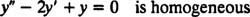butThe nonhomogeneous equation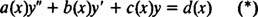can be turned into a homogeneous one simply by replacing the right‐hand side by 0: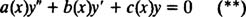Equation (**) is called the homogeneous equation corresponding to the nonhomogeneous equation, (*). There is an important connection between the solution of a nonhomogeneous linear equation and the solution of its corresponding homogeneous equation. The two principal results of this relationship are as follows:

Theorem A. If y 1( x) and y 2( x) are linearly independent solutions of the linear homogeneous equation (**), then every solution is a linear combination of y 1 and y 2. That is, the general solution of the linear homogeneous equation is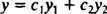Theorem B. If y( x) is any particular solution of the linear nonhomogeneous equation (*), and if y h ( x) is the general solution of the corresponding homogeneous equation, then the general solution of the linear nonhomogeneous equation is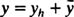That is,[Note: The general solution of the corresponding homogeneous equation, which has been denoted here by y h , is sometimes called the complementary function of the nonhomogeneous equation (*).] Theorem A can be generalized to homogeneous linear equations of any order, while Theorem B as written holds true for linear equations of any order. Theorems A and B are perhaps the most important theoretical facts about linear differential equations—definitely worth memorizing.

Example 1: The differential equation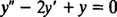is satisfied by the functionsVerify that any linear combination of y 1 and y 2 is also a solution of this equation. What is its general solution?

Every linear combination of y 1 = e x and y 2 = xe x looks like this:for some constants c 1 and c 2. To verify that this satisfies the differential equation, just substitute. If y = c 1 e x + c 2 xe x , thenSubstituting these expressions into the left‐hand side of the given differential equation givesThus, any linear combination of y 1 = e x and y 2 = xe x does indeed satisfy the differential equation. Now, since y 1 = e x and y 2 = xe x are linearly independent, Theorem A says that the general solution of the equation is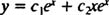Example 2: Verify that y = 4 x – 5 satisfies the equationThen, given that y 1 = e x and y 2 = e 4x are solutions of the corresponding homogeneous equation, write the general solution of the given nonhomogeneous equation.

First, to verify that y = 4 x – 5 is a particular solution of the nonhomogeneous equation, just substitute. If y = 4 x – 5, then y′ = 4 and y″ = 0, so the left‐hand side of the equation becomes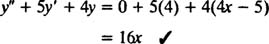Now, since the functions y 1 = e x and y 2 = e 4x are linearly independent (because neither is a constant multiple of the other), Theorem A says that the general solution of the corresponding homogeneous equation is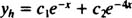Theorem B then saysis the general solution of the given nonhomogeneous equation.

Example 3: Verify that both y 1 = sin x and y 2 = cos x satisfy the homogeneous differential equation y″ + y = 0. What then is the general solution of the nonhomogeneous equation y″ + y = x?

If y 1 = sin x, then y1 + y 1 does indeed equal zero. Similarly, if y 2 = cos x, then y2 = y is also zero, as desired. Since y 1 = sin x and y 2 = cos x are linearly independent, Theorem A says that the general solution of the homogeneous equation y″ + y = 0 isNow, to solve the given nonhomogeneous equation, all that is needed is any particular solution. By inspection, you can see that y = x satisfies y″ + y = x. Therefore, according to Theorem B, the general solution of this nonhomogeneous equation is